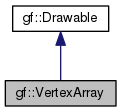Gamedev Framework (gf)  0.4.0 A C++11 framework for 2D games
gf::VertexArray Class Reference

A set of primitives. More...

#include <gf/VertexArray.h>

Inheritance diagram for gf::VertexArray:[legend]

## Public Member Functions

VertexArray ()
Default constructor. More...

VertexArray (PrimitiveType type, std::size_t count=0)
Construct the vertex array with a type and an initial number of vertices. More...

std::size_t getVertexCount () const
Return the vertex count. More...

const VertexgetVertexData () const
Return the vertex data. More...

Vertexoperator[] (std::size_t index)
Get a read-write access to a vertex by its index. More...

const Vertexoperator[] (std::size_t index) const
Get a read-only access to a vertex by its index. More...

bool isEmpty () const
Check if the vertex array is empty. More...

void clear ()
Clear the vertex array. More...

void resize (std::size_t count)
Resize the vertex array. More...

void reserve (std::size_t capacity)
Increase the capacity of the vertex array. More...

void append (const Vertex &vertex)
Add a vertex to the array. More...

void setPrimitiveType (PrimitiveType type)
Set the type of primitives to draw. More...

PrimitiveType getPrimitiveType () const
Get the type of primitives drawn by the vertex array. More...

RectF getBounds () const
Compute the bounding rectangle of the vertex array. More...

virtual void draw (RenderTarget &target, RenderStates states) override
Draw the object to a render target. More...Public Member Functions inherited from gf::Drawable
virtual ~Drawable ()
Virtual desctructor. More...

## Detailed Description

A set of primitives.

gf::VertexArray is a very simple wrapper around a dynamic array of vertices and a primitive type.

It inherits gf::Drawable, but unlike other drawables it is not transformable.

Example:

gf::VertexArray lines(gf::PrimitiveType::LinesStrip, 4);
lines.position = gf::Vector2f(10, 0);
lines.position = gf::Vector2f(20, 0);
lines.position = gf::Vector2f(30, 5);
lines.position = gf::Vector2f(40, 2);
window.draw(lines);
gf::Vertex

## Constructor & Destructor Documentation

 gf::VertexArray::VertexArray ( )
inline

Default constructor.

Creates an empty vertex array. The default primitive type is gf::PrimitiveType::Points.

 gf::VertexArray::VertexArray ( PrimitiveType type, std::size_t count = 0 )
inline

Construct the vertex array with a type and an initial number of vertices.

Parameters
 type Type of primitives count Initial number of vertices in the array

## Member Function Documentation

 void gf::VertexArray::append ( const Vertex & vertex )
inline

Add a vertex to the array.

Parameters
 vertex The vertex to add
 void gf::VertexArray::clear ( )
inline

Clear the vertex array.

This function removes all the vertices from the array. It doesn't deallocate the corresponding memory, so that adding new vertices after clearing doesn't involve reallocating all the memory.

 virtual void gf::VertexArray::draw ( RenderTarget & target, RenderStates states )
overridevirtual

Draw the object to a render target.

This is a pure virtual function that has to be implemented by the derived class to define how the drawable should be drawn.

Parameters
 target Render target to draw to states Current render states

Implements gf::Drawable.

 RectF gf::VertexArray::getBounds ( ) const

Compute the bounding rectangle of the vertex array.

This function returns the minimal axis-aligned rectangle that contains all the vertices of the array.

Returns
Bounding rectangle of the vertex array
 PrimitiveType gf::VertexArray::getPrimitiveType ( ) const
inline

Get the type of primitives drawn by the vertex array.

Returns
Primitive type
 std::size_t gf::VertexArray::getVertexCount ( ) const
inline

Return the vertex count.

Returns
Number of vertices in the array
 const Vertex* gf::VertexArray::getVertexData ( ) const
inline

Return the vertex data.

Returns
A pointer to the vertices in the array
 bool gf::VertexArray::isEmpty ( ) const
inline

Check if the vertex array is empty.

Returns
True if the vertex array is empty
 Vertex& gf::VertexArray::operator[] ( std::size_t index )
inline

Get a read-write access to a vertex by its index.

This function doesn't check index, it must be in range $$[0, n - 1]$$ where $$n$$ is the vertex count. The behavior is undefined otherwise.

Parameters
 index Index of the vertex to get
Returns
Reference to the index-th vertex
getVertexCount()
 const Vertex& gf::VertexArray::operator[] ( std::size_t index ) const
inline

Get a read-only access to a vertex by its index.

This function doesn't check index, it must be in range $$[0, n - 1]$$ where $$n$$ is the vertex count. The behavior is undefined otherwise.

Parameters
 index Index of the vertex to get
Returns
Const reference to the index-th vertex
getVertexCount()
 void gf::VertexArray::reserve ( std::size_t capacity )
inline

Increase the capacity of the vertex array.

Parameters
 capacity New capacity of the array
 void gf::VertexArray::resize ( std::size_t count )
inline

Resize the vertex array.

If count is greater than the current size, the previous vertices are kept and new (default-constructed) vertices are added.

If count is less than the current size, existing vertices are removed from the array.

Parameters
 count New size of the array (number of vertices)
 void gf::VertexArray::setPrimitiveType ( PrimitiveType type )
inline

Set the type of primitives to draw.

This function defines how the vertices must be interpreted when it's time to draw them:

• As points
• As lines
• As triangles

The default primitive type is gf::PrimitiveType::Points.

Parameters
 type Type of primitive